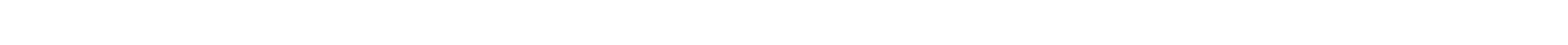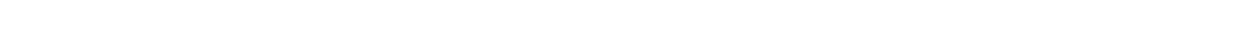X

Choose an account type

# Annualized Rate of Return Calculator# Annualized Rate of Return Calculator

Our free Annualized Rate of Return Calculator determines the rate of return on a compounded-interest investment or account.

What is an annualized rate of return?

Creditor-debtor agreement terms may vary but they all include a requirement to compare dividend rates, also called rates of return. Annualization does that by calculating an equivalent annual dividend rate from a given dividend rate and a non-annual compounding time period. This time period is also expressed in terms of “periods per year,” and refers to how often a given rate is applied and calculated to compounded-interest investments.

### Dividend Rate

The dividend rate specifies what percentage of an invested amount is paid to the creditor at regular time intervals. In this Annualized Rate of Return Calculator, the dividend rate is entered exactly as a percentage numeric value.